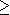# OEF Sequences --- Introduction ---

This module actually contains 16 exercises on infinite sequences: convergence, limit, recursive sequences, ...

### Two limits

Let () be an infinite sequence of real numbers. If one has

and for ,

what can be said about its convergence? (You should choose the most pertinent consequence.)

### Comparison of sequences

Let () and () be two sequences of real numbers where () converges towards . If one has

,

what can be said about the convergence of ()? (You must choose the most pertinent consequence.)

### Growth and bound

Let () be a sequence of real numbers. If () is , what can be said about its convergence (after its existence)?

### Convergence and difference of terms

Let be a sequence of real numbers. Among the following assertions, which are true, which are false?
1. If , then .

2. If , then .

### Convergence and ratio of terms

Let be a sequence of real numbers. Among the following assertions, which are true, which are false?
1. If , then .

2. If , then .

### Epsilon

Let be a sequence of real numbers. What does the condition

imply on the convergence of ? (You must choose the most pertinent consequence.)

### Fraction 2 terms

Compute the limit of the sequence (un), where

### Fraction 3 terms

Compute the limit of the sequence (un), where

### Fraction 3 terms II

Compute the limit of the sequence (un), where

WARNING IN this exercise, approximative replies will be considered as false! Type pi instead of 3.14159265, for example.

### Growth comparison

What is the nature of the sequence (un), where

?

### Monotony I

Study the growth, sup, inf, min, max of the sequence (un) for n, where

.

Write for a value that does not exist, and or - for +or -.

### Monotony II

Study the growth, sup, inf, min, max of the sequence (un) for n, where

.

Write for a value that does not exist, and or - for +or -.

### Powers I

Compute the limit of the sequence (un), where

### Powers II

Compute the limit of the sequence (un), where

Type no if the sequence is divergent.

### Recursive function

The sequence such that
is a recursive sequence defined by for a certain function . Find this function.

### Recursive limit

Find the limit of the recursive sequence such that
In order to access WIMS services, you need a browser supporting forms. In order to test the browser you are using, please type the word wims here: and press Enter''.